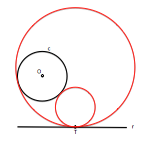# Metric geometry : Investment : Application to the resolution of problems and angular tangentsThe investment It is a transformation that allows to solve problems with angular conditions.

It can be applied directly or used to reduce other problems addressed simplest known nature.

The different approaches with which we can deal with a problem will be studied by developing a simple classic problem of tangents.

The generalization of the ideas discussed other forms of statement, similar problems of the same nature, an exercise that will allow the reader to systematize solving models.

## Problem studied

Consider the following problem:

Determining circumferences are tangent to a circle and a straight line in one of its points.

The problem may be a particular case of angularity, particularly isogonalidad (angle equal), respect to two circles and a condition of step. Three aspects of this case without subtracting simplify generality:

• The angle can be considered null (condition tangencias).
• One of the circles is a line (radio infinity)
• The crossing point is located on one of the elements (Nip T)

These singularities are simplify plotting (number of lines needed to resolve) although the terms used are the same. The utility in a didactic problem is precisely that simplification since it allows focusing thoughts with less difficulty.

This problem could be stated generally as:

Determine the circumference forming alpha and beta angles with two given circles and passing through a point P.

Analysis model will resolve as the first case later with comments necessary for the reader to address the generic case and, accordingly, full range of cases arising.

## Focus first: Simpler solution sought

We first address the problem by less conceptual and more laborious approach from the point of view of graphic layouts necessary. This model shall apply subject to the availability of a waypoint or as a condition for geometric constraint problem, not allowing the generalization to the case of three circles. It is therefore a great application incomplete although numerous problems approach.

Investment will apply to the dataset, resolve the problem with the data processed and undoing the transformation ( the solution obtained in the inverted set) determine the solution sought.

In this model solution we will use the crossing point as center of inversion. Doing this and transform data the solution we seek will become a simpler geometric element (a line), greatly simplifying the problem.

The main idea is to simplify the solution thus find

The power value may be any, including some items that transform himself drawn to simplify. At one level of analysis can avoid these particular values ​​of the investment power to clearly differentiate the original set and the transformed.

The point P and Q cutting the circumference doubles chosen self-inverting. Be transformed circumference tangent to the tangent t1 and t2 investment from the center to the circumference, as we saw when studying the Investment in the plane.

On the investment power power point T about the circumference c, it becomes a double circle (orthogonal to the self-inverting).

The line r is reverse itself, and passing through the center of inversion.

As the desired circumference through the point T we have taken as investment hub, its transform is a line that does not pass through this point, and comply with the respective angular conditions (tangency) respect of the inverses of the circumference c and the line r ( c is tangent to’ y a r’ ).

The condition of tangency between the straight condition results in parallelism between them.

In the figure has been obtained the solution transforms, as described.

The straight s'2 s'1 and become solutions to the problem by undoing the transformation. The points of tangency of these lines will become the tangent of these solutions.

If instead of having tangency conditions we had angular conditions, tangent lines s'2 s'1 and the circles they would goniómetras we determined to study the problems of straight with angular conditions.

## Second approach: Reversing a data in another

This approach is more general, allowing to reduce complex problems to fundamental problem for the case straight tangents or circumference, or obtain relationships between the elements that simplify.

We use two investment centers that relate to the line r and circumference c ( the circles of the). A positive center I , and one that will have negative power, I-. Analysis in this case on the circumference must encontrase c.

The nip T is transformed into T’ through investment and positive power T” investment with negative power, causing each one of the solutions searched.

Under these conditions any element tangent to the circumference c has become one tangent to the transformed, The straight r=c’. The solutions will therefore double circles, reverse themselves, that pass through the points T and T’ and be orthogonal to the self-inverting (not shown)

Solutions are determined to find their centers in the perpendicular to the line through the point of tangency, in the perpendicular TT’ or aligned with the center of the circle to its data nip.

In another article for angularity generalize Generic; see the orthogonality condition that the circumference can reduce a self-inverting beams solutions circumferences families.

This investment approach of a data into another is the basis for the replacement of angular conditions for orthogonality conditions.

Metric geometry